﻿ 水下航行器电池舱热响应仿真分析
 舰船科学技术2020, Vol. 42Issue (8): 109-114    DOI: 10.3404/j.issn.1672-7649.2020.08.021PDF

The battery cabin thermal response simulation analysis of underwater vehicle
GUO Jun, ZHANG Kai, LIU Jia
The 705 Research Institute of CSSC, Xi′an 710077, China
Abstract: Battery cabin of electric underwater vehicle works in a bad environment. The influence of battery heat dissipation on the overall structure must be considered in the design to avoid structural damage due to excessive local stress. Firstly, the mathematical model of thermal conduction, radiation and convection analysis for a certain type of underwater vehicle battery cabin is established, and the finite element model is constructed based on the finite element simulation software Ansys. Under the corresponding thermal load, the temperature distribution of the compartment, the maximum deformation and stress at the installation plate are analyzed. The results verifies the rationality of the structural design. The research method in this paper has good universality, and the structural design has reference significance for the structural layout of underwater vehicle battery compartment.
Key words: battery cabin     thermal analysis     finite element     stress
0 引　言

1 数学模型 1.1 热传导

 $\rho c\frac{{\partial t}}{{\partial \tau }} = \frac{\partial }{{\partial x}}\left( {\lambda \frac{{\partial t}}{{\partial x}}} \right) + \frac{\partial }{{\partial y}}\left( {\lambda \frac{{\partial t}}{{\partial y}}} \right) + \frac{\partial }{{\partial z}}\left( {\lambda \frac{{\partial t}}{{\partial z}}} \right) + \dot \varPhi\text{。}$ (1)

1.2 热对流

 $\varPhi = hA({T_S} - {T_F})\text{。}$ (2)

 $Re = \frac{{ul}}{\nu } = 1.0 \times {10^6}\text{。}$ (3)

 $N{u_l} = 0.332{Re^{1/2}}{Pr^{1/3}} = 6.48 \times {10^2}({\rm{W}}/{{\rm{m}}^2} \cdot {\rm{K}}) \text{。}$ (4)

 ${h_l} = \frac{{N{u_l} \cdot \lambda }}{l} = 1\;200({\rm{W}}/{{\rm{m}}^2} \cdot {\rm{K}}) \text{。}$ (5)

1.3 辐射换热

 $Q = \sigma \varepsilon {A_i}{F_{ij}}(T_i^4 - T_j^4)\text{。}$ (6)

2 有限元仿真模型 2.1 物理模型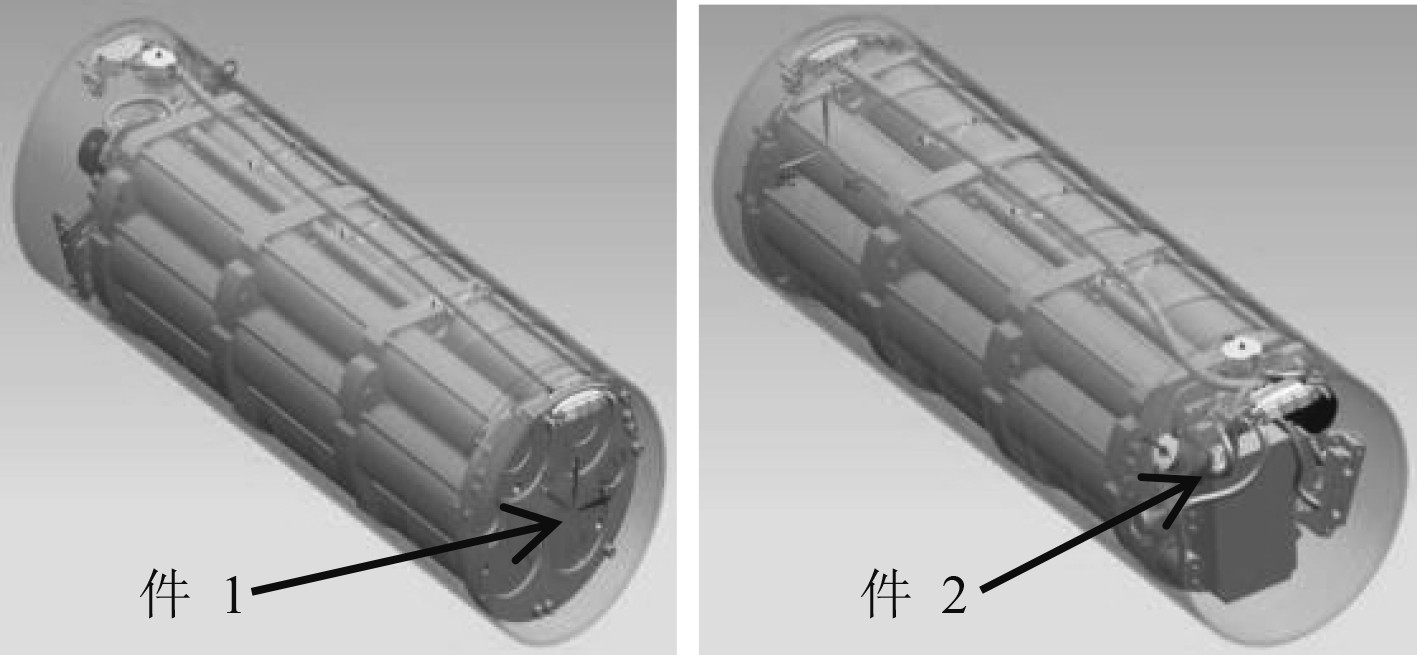图 1 舱段模型装配示意图 Fig. 1 Battery compartment assembly drawing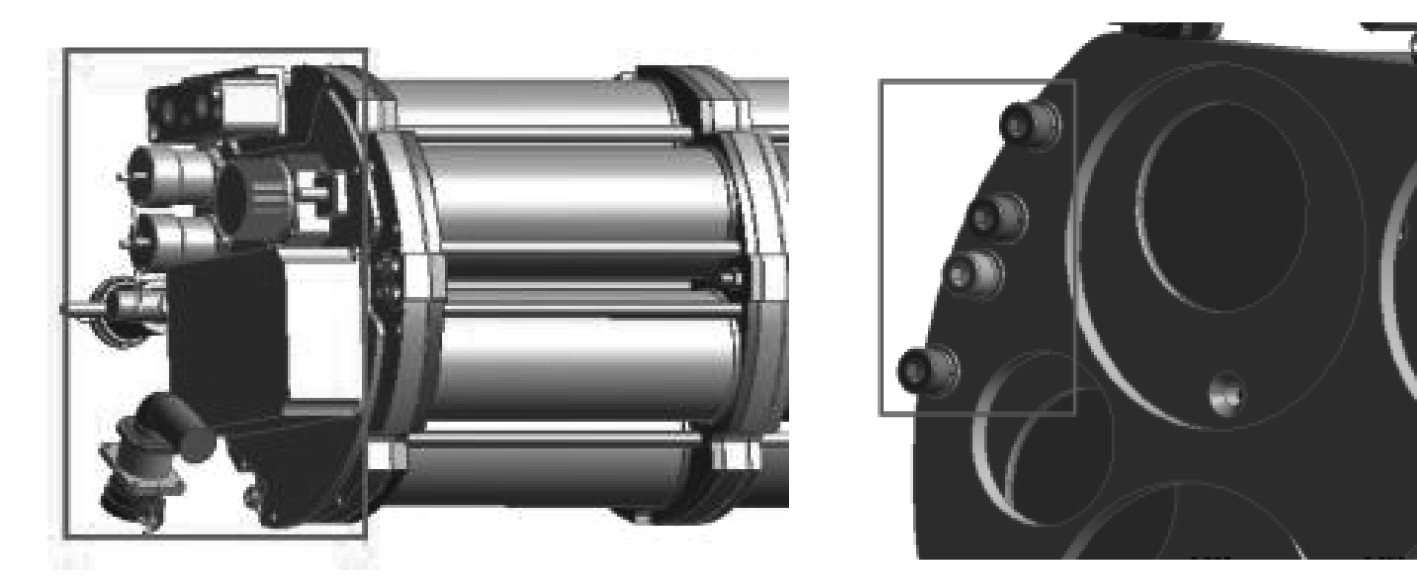图 2 原始模型 Fig. 2 Original modal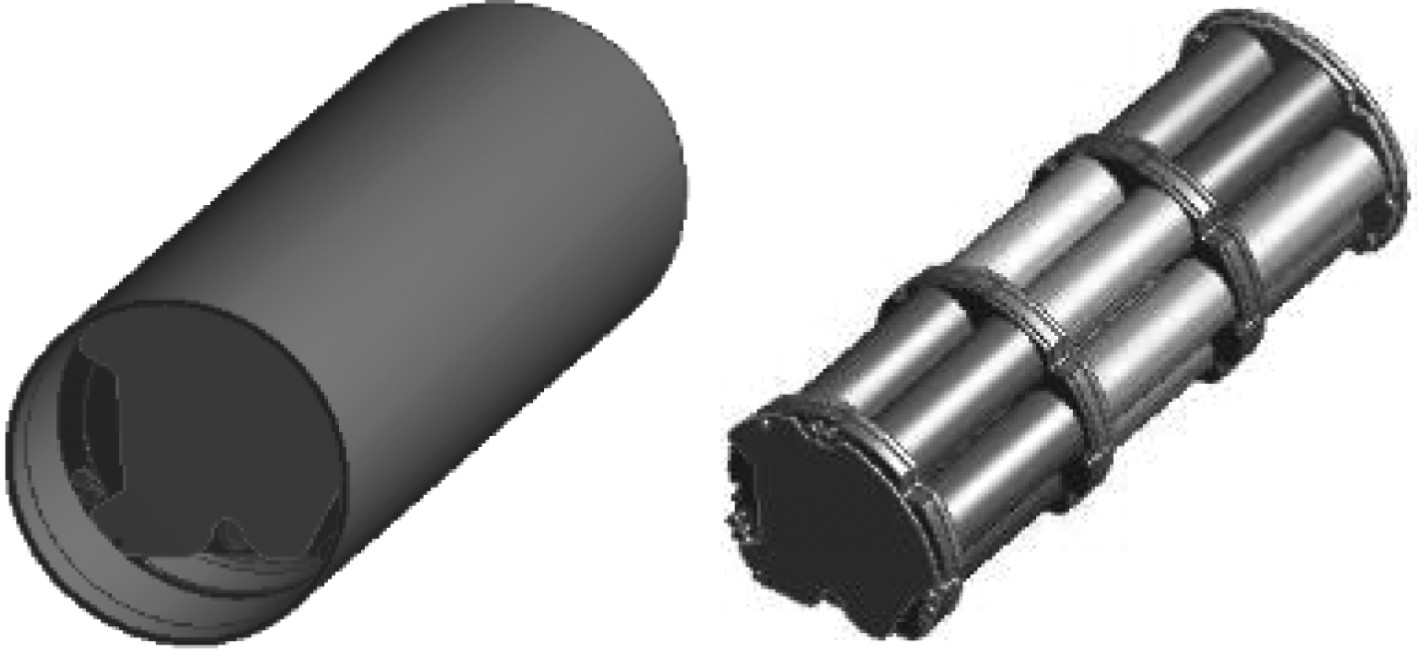图 3 整体简化整体 Fig. 3 Overall simplified modal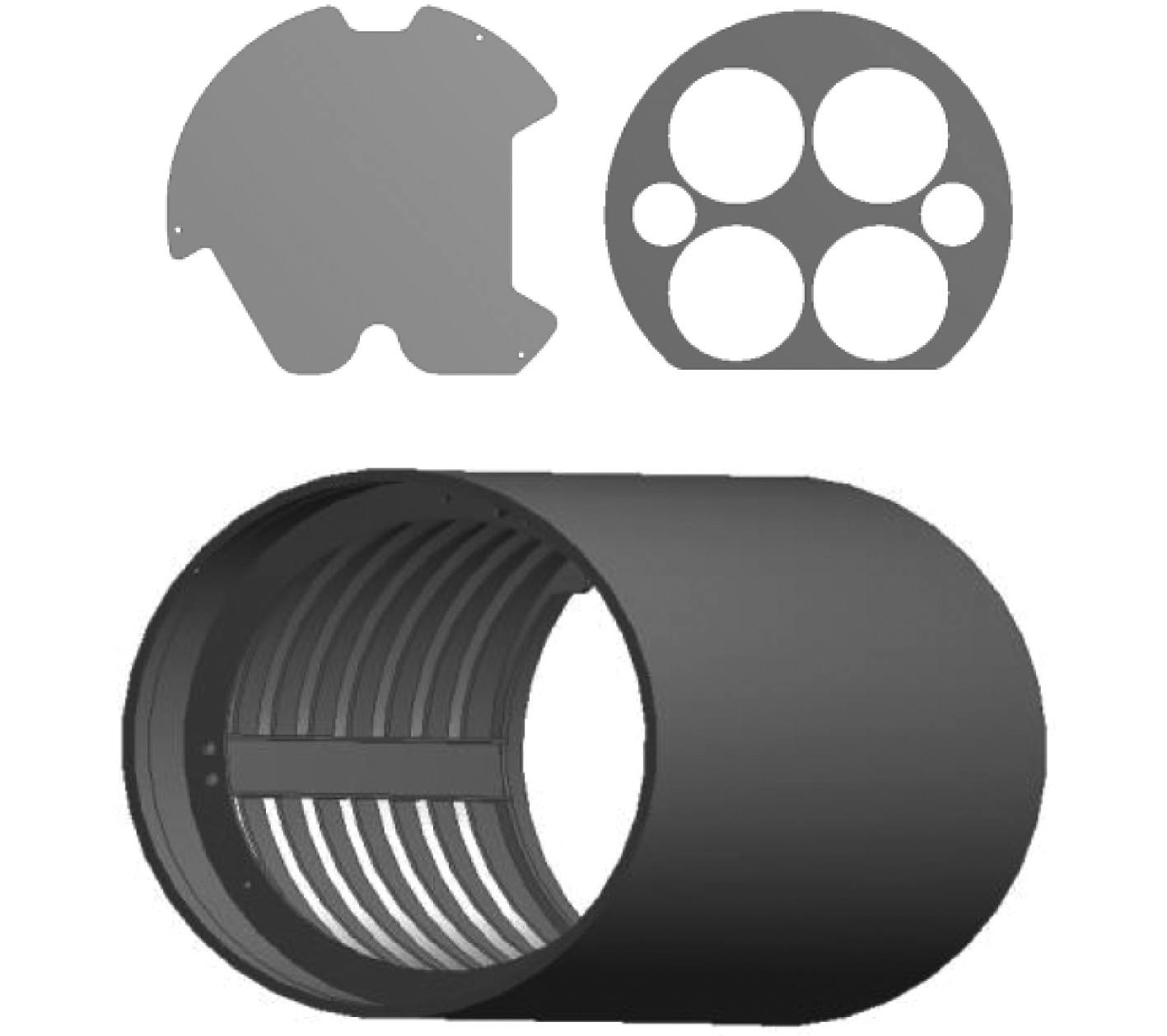图 4 简化后局部模型 Fig. 4 Locally simplified model
2.2 网格划分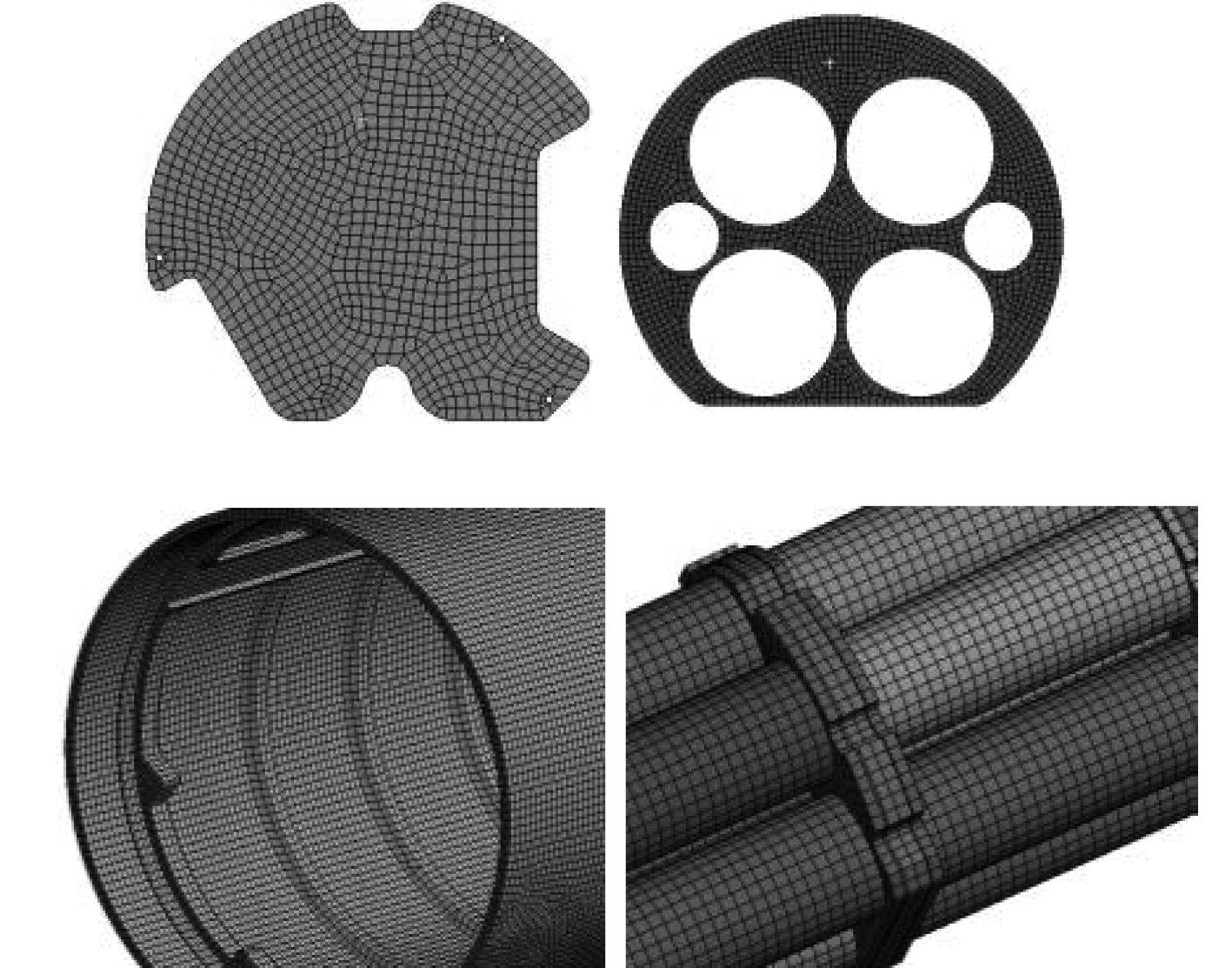图 5 网格划分细节 Fig. 5 The mesh detials
2.3 接触关系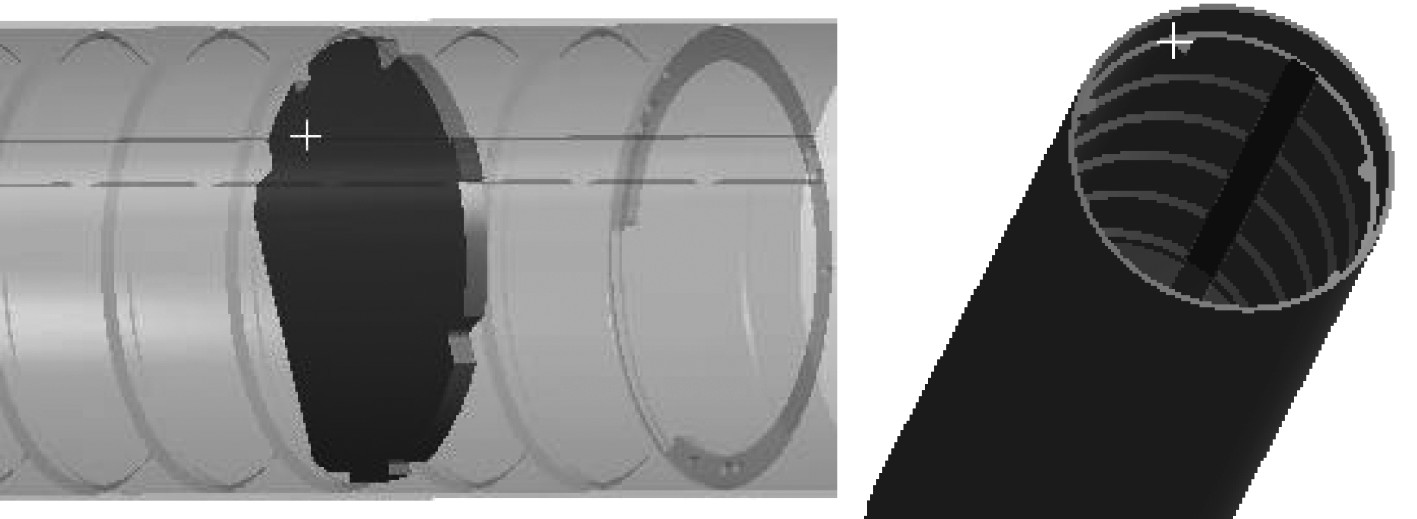图 6 中间安装板与壳体接触对（No Separation） Fig. 6 Contact pair between the middle plate and the shell （No Separation）
2.4 材料属性表 1 铝合金材料属性 Tab.1 Properties of aluminum alloy表 4 螺钉材料属性 Tab.4 Properties of screw表 5 应力分析材料属性 Tab.5 Material properties of the stress analysis表 2 钛合金材料属性 Tab.2 Properties of titanim alloy表 3 垫片材料属性 Tab.3 Properties of shim
2.5 边界条件及载荷设置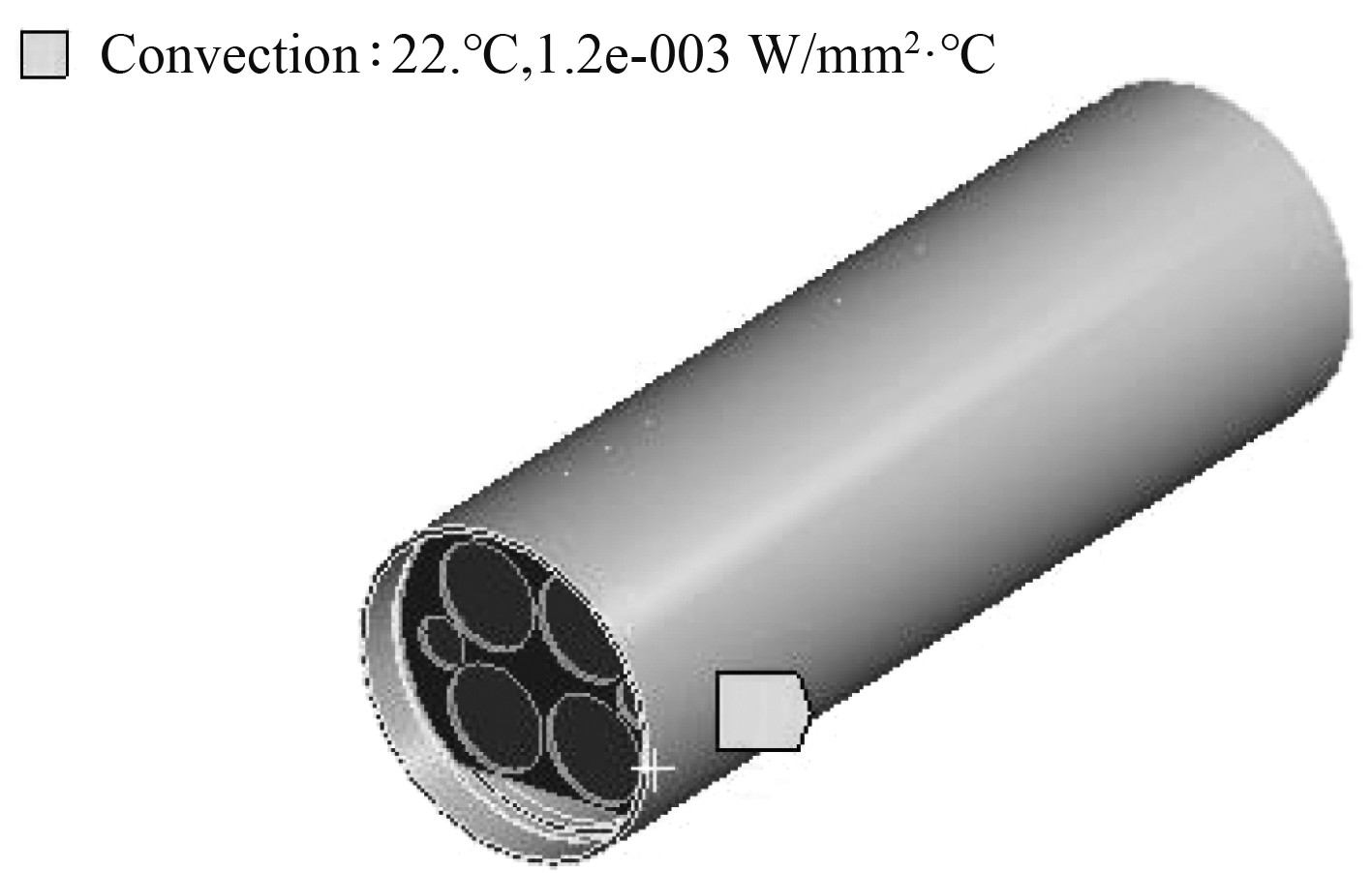图 7 对流换热边界条件 Fig. 7 Boundary conditions for convective heat transfer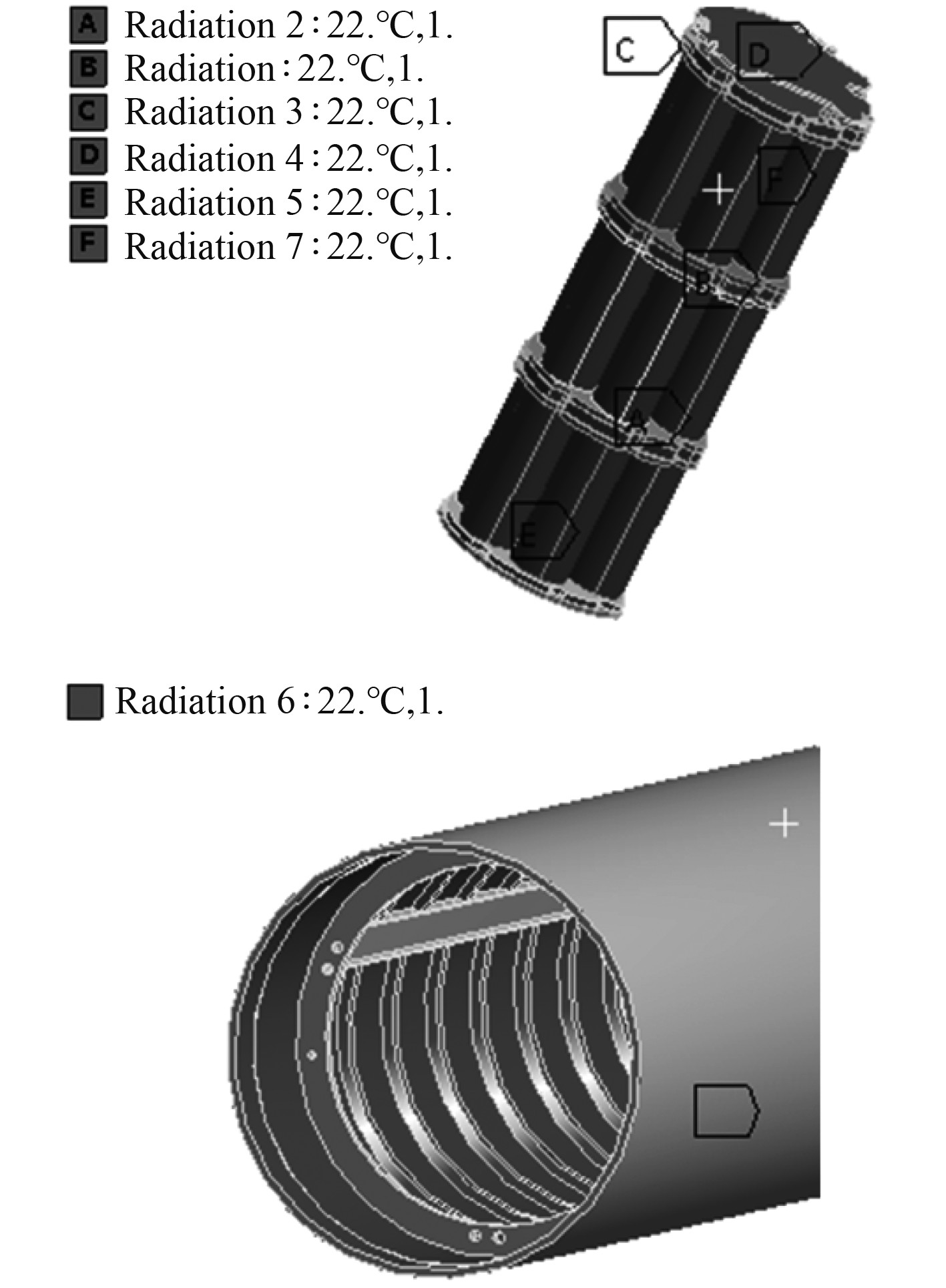图 8 辐射换热边界条件 Fig. 8 Boundary conditions for radiative heat transfer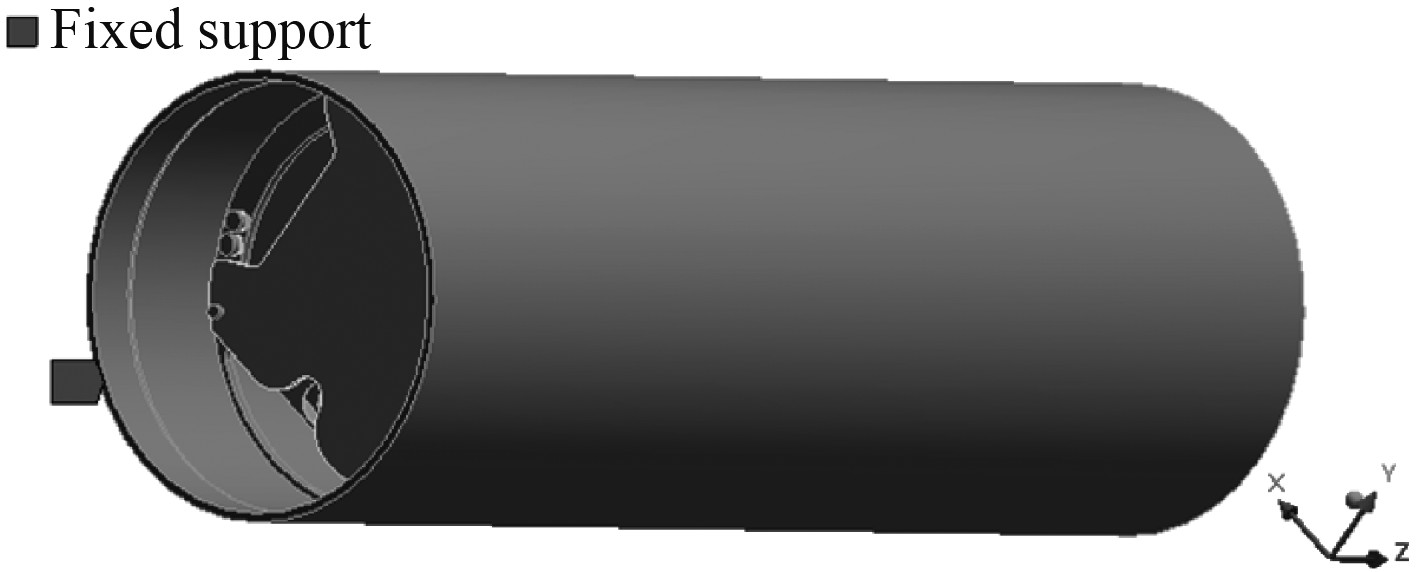图 9 约束条件 Fig. 9 Displacement constraints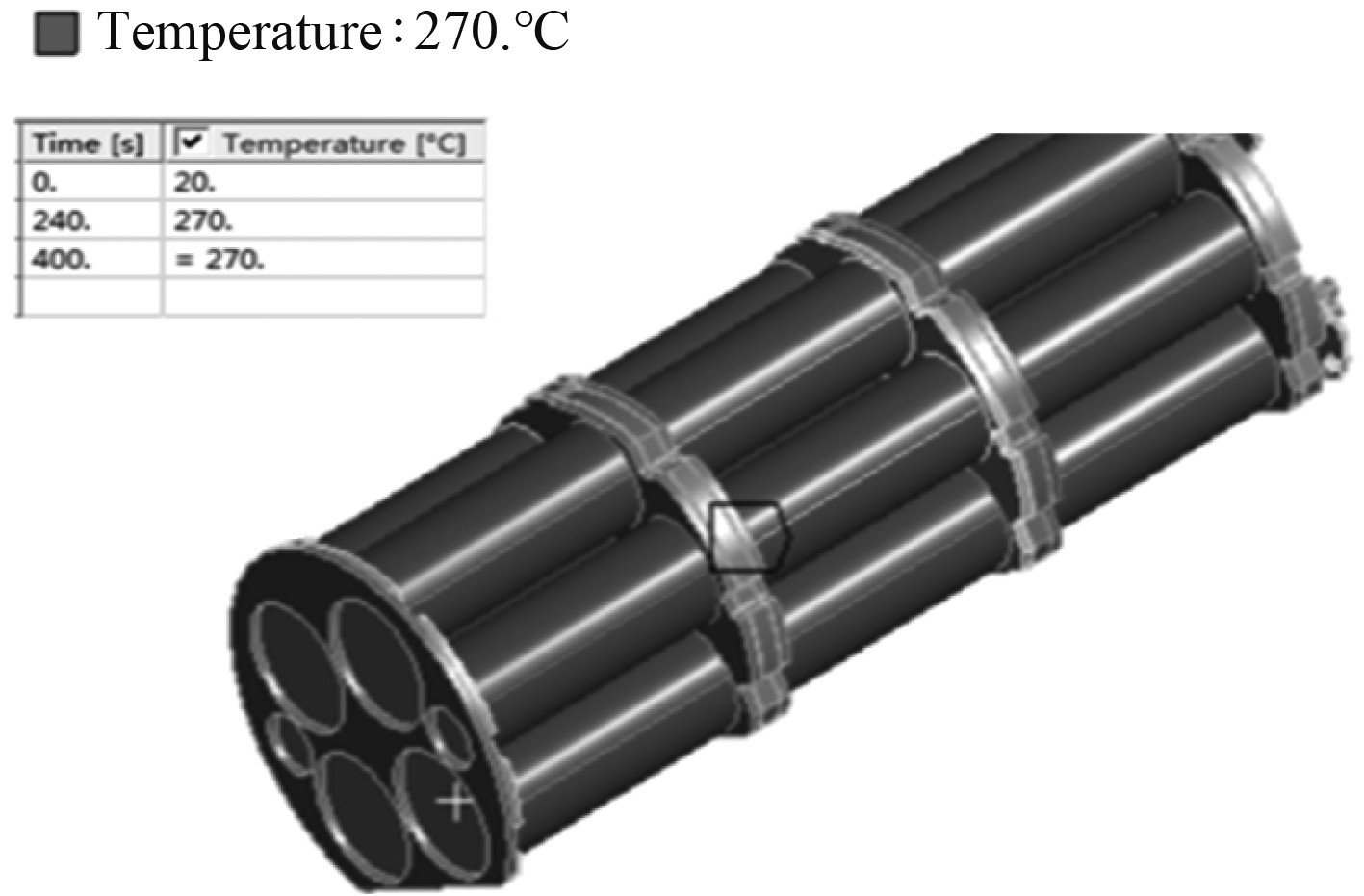图 10 温度载荷 Fig. 10 Temperture load
3 仿真结果及分析 3.1 温度分析结果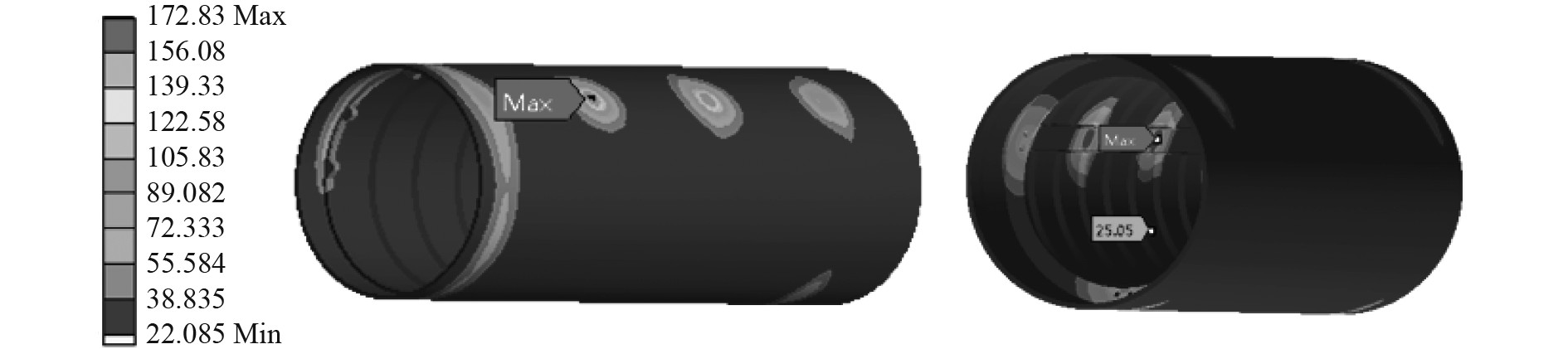图 11 温度分布云图 Fig. 11 Temperature distribution nephogram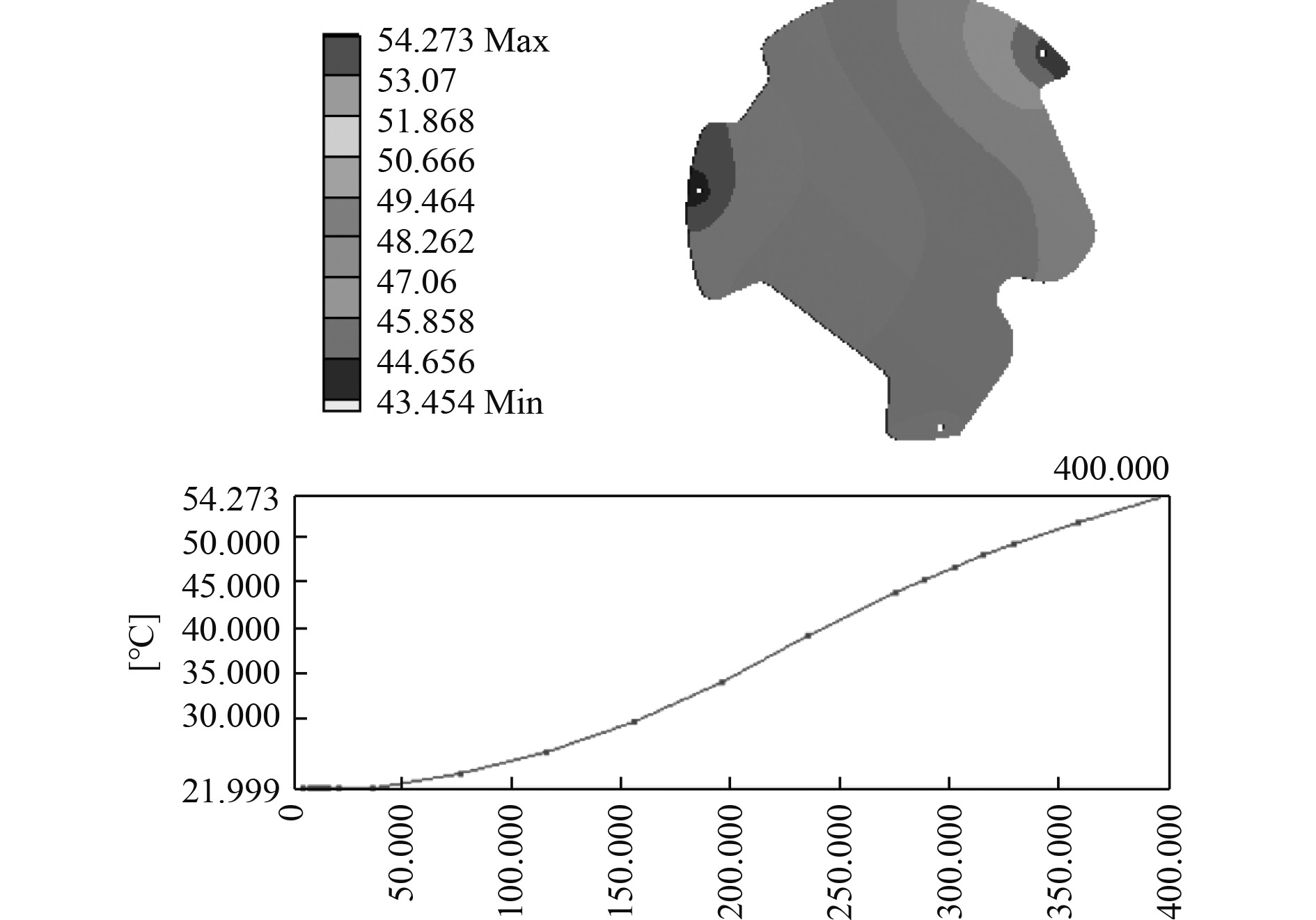图 12 前安装板温度分布云图 Fig. 12 Temperture distribution nephogram of front plate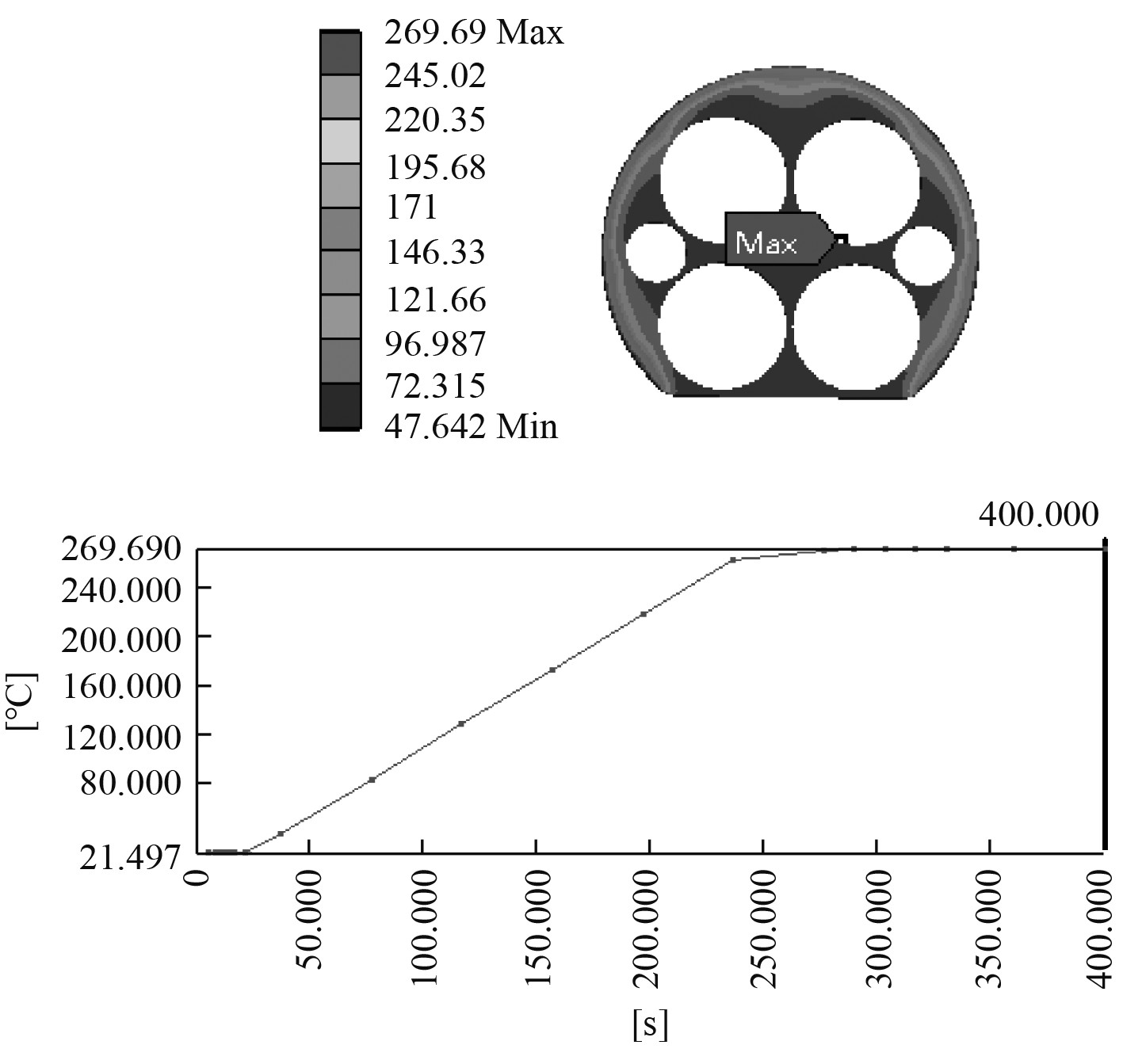图 13 后安装板温度分布云图 Fig. 13 Temperture distribution nephogram of rear plate

3.2 变形及应力分析结果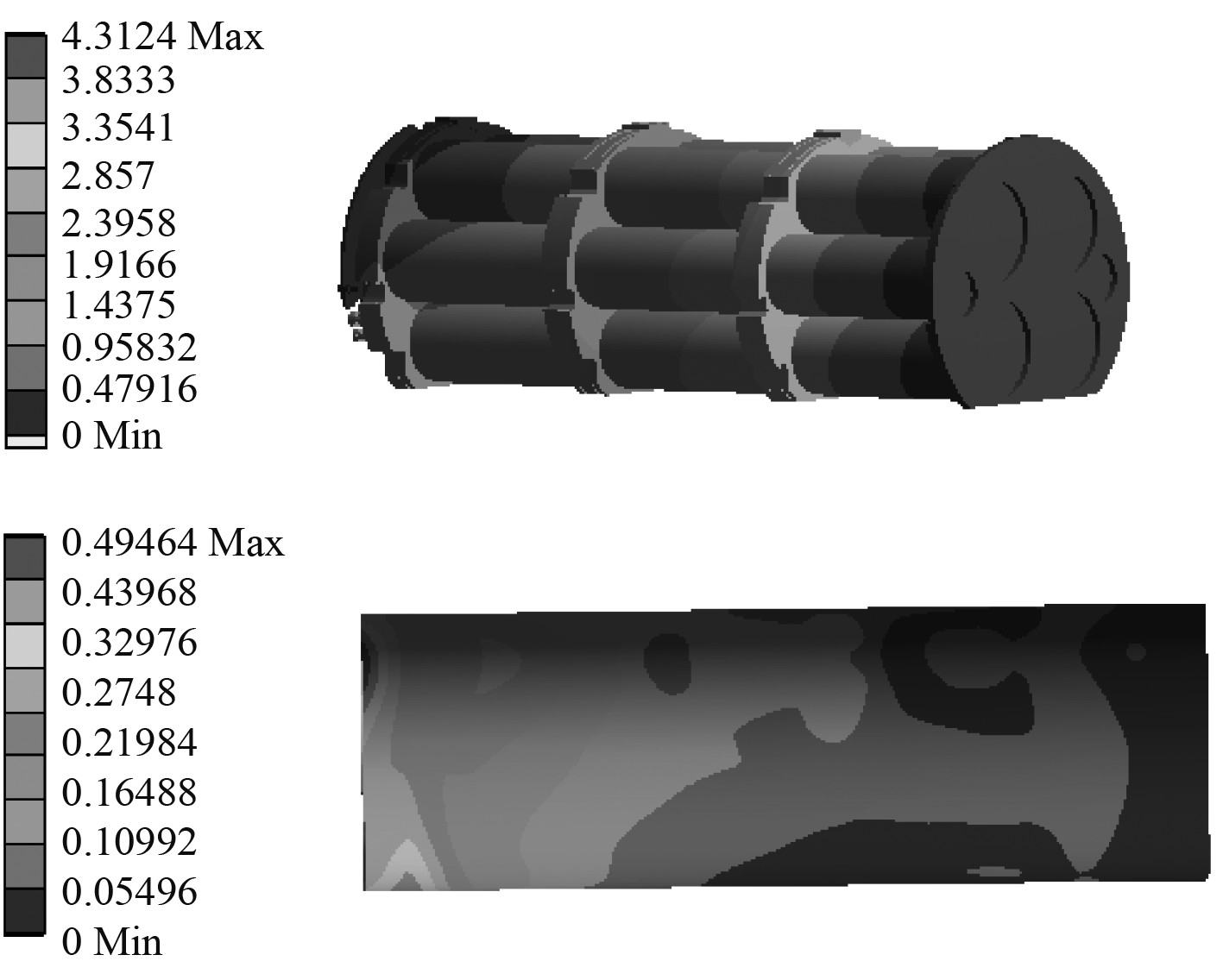图 14 模型变形云图 Fig. 14 The deformation nephogram图 15 前后安装板变形云图 Fig. 15 The deformation nephogram of plates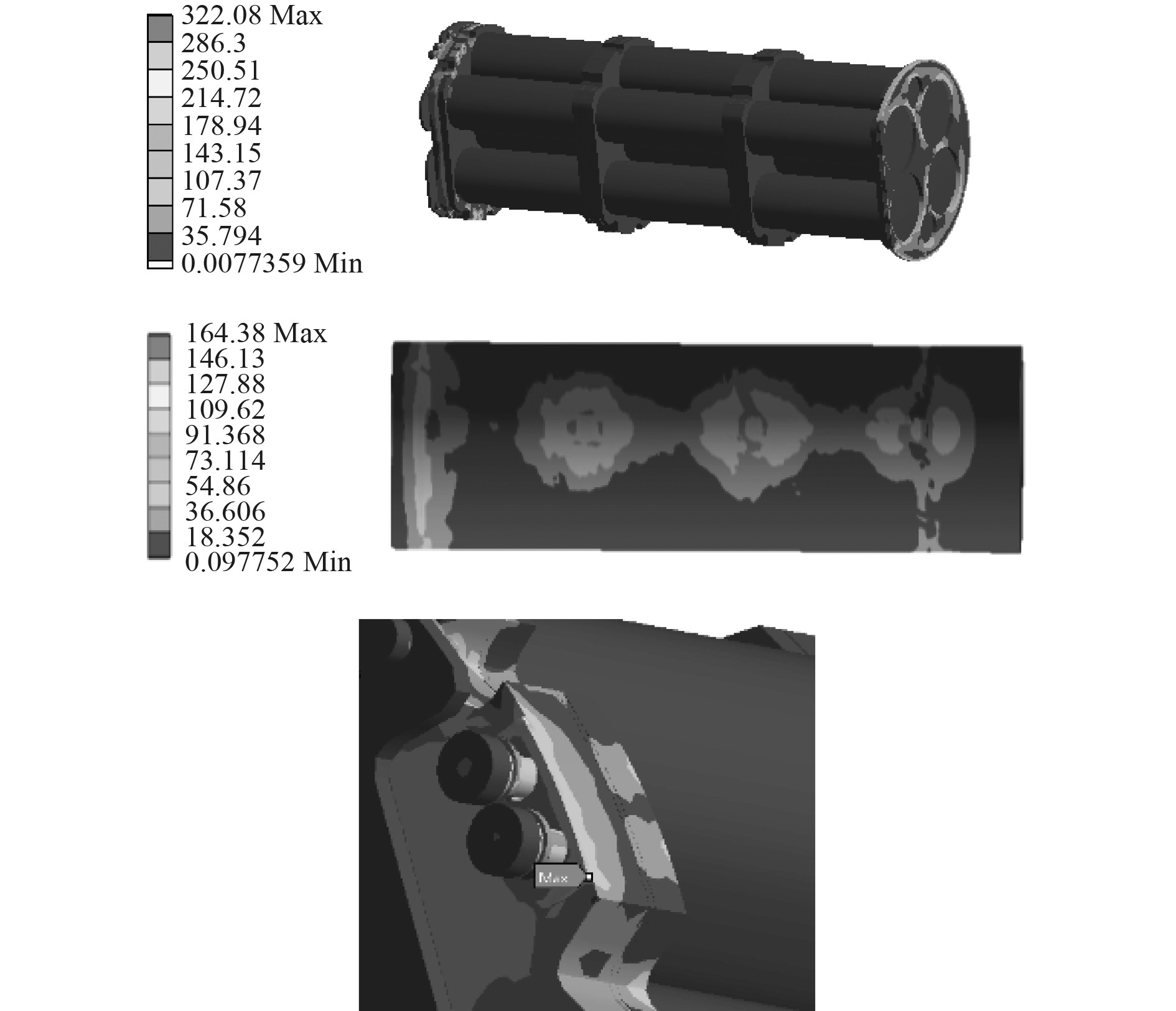图 16 模型应力分布云图 Fig. 16 Stress distribution nephogram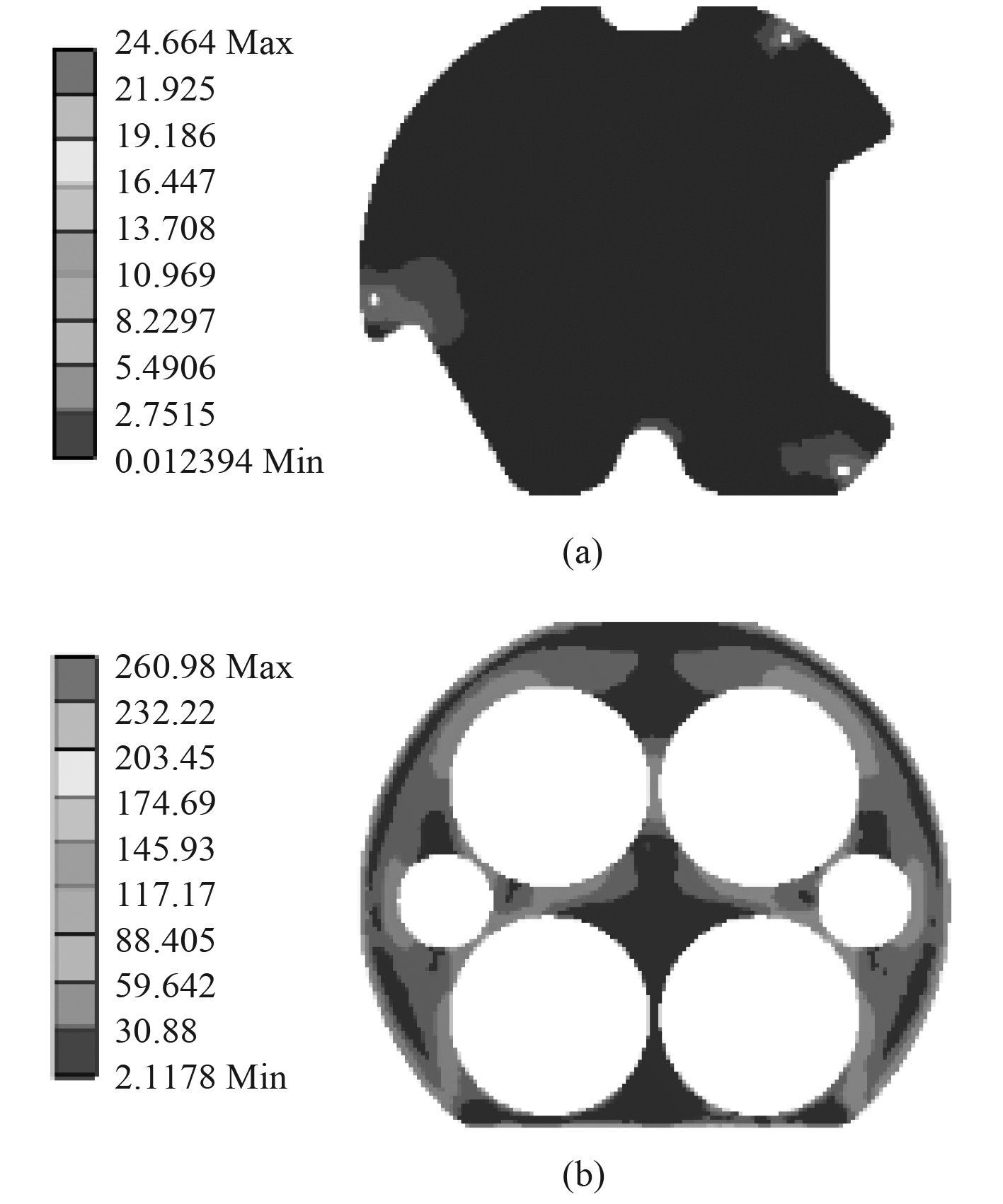图 17 前后安装板应力云图 Fig. 17 Stress distribution nephogram of plates
4 结　语

1）舱段瞬态温度场分析结果表明, 壳体在400 s时温度最高是172 ℃，前安装板的最高温度为54 ℃，后安装板的最高温度为269 ℃。

2）舱段应力场计算结果表明，舱段整体最大变形量为4.3 mm，在结构设计时，要充分考虑这个热变形量，否则会因热拘束而导致结构某些部位出现大的热应力。

3）本次计算的热应力最大为322 MPa，出现在安装螺栓的小凸台的顶角位置，属于典型的应力集中现象，但此处不属于结构关心区域，且其余各部分应力幅值均小于各部分材料的屈服强度，因此舱段整体结构的强度满足要求，结构安全。

  蔡年生. UUV动力电池现状及发展趋势[J]. 水下无人系统学报, 2010, 18(2): 81-87. DOI:10.3969/j.issn.1673-1948.2010.02.001  李尉, 宋保维, 胡欲立. 密闭电池舱段温度场数值仿真[J]. 水下无人系统学报, 2011, 19(4). DOI:10.3969/j.issn.1673-1948.2011.04.018  郝文广, 王建平, 王志强. 聚合物锂离子电池大倍率放电温度场分析[J]. 水下无人系统学报, 2013, 21(5): 360-363.  王艳峰, 胡欲立. 电池架导热系数对水下航行器电池舱段内部发热和传热过程的影响[J]. 上海: 上海交通大学学报, 2012, 46(5): 683-687.  王晋鹏, 王小博, 牛惜晨. 密闭电池舱段内锂离子电池组温度场分析[J]. 电源技术, 2010, 34(12): 1233-1235. DOI:10.3969/j.issn.1002-087X.2010.12.006  杨世铭. 传热学. 第4版 [M]. 北京: 高等教育出版社, 2006.